# CosmoPhotoz Trial Run

Version 1.0, Bart Buelens, 4 November 2014.

www.astrostatistics.org

## Introduction

We explore the possibilities of the recently released R package CosmoPhotoz. The package contains functions facilitating the modeling of redshifts as a function of photometric data for multi-wavelength observations of galaxies. The models under consideration are Generalised Linear Models (GLMs). The dependent variable is the redshift (z), which when estimated from photometric data is commonly referred to as photo-z. The independent variables or predictors in the models are magnitudes measured in different wavelength bands.

We largely follow the tutorial provided by the CosmoPhotoz authors at http://rafaelsdesouza.github.io/CosmoPhotoz/, and elaborate in several ways.

The R package CosmoPhotoz contains functions that allow an implementation of the procedure discussed by Elliott, de Souza, Krone-Martins, Cameron, Ishida, Hilbe in their paper “The Overlooked Potential of Generalized Linear Models in Astronomy-II: Gamma regression and photometric redshifts” (2014), available at http://arxiv.org/abs/1409.7699.

While the paper presents results on both a simulated and a real data set, only the simulated data set is included in the package. We refer the reader to the paper for further details on the data and the methods.

On CRAN, the package is available at http://cran.r-project.org/web/packages/CosmoPhotoz/index.html.

The present document is produced using R v. 3.0.2 and CosmoPhotoz v. 0.1.

Assuming the package has been installed, it is loaded as follows, together with some additional libraries that we need further down.

``````library(CosmoPhotoz)
library(ggplot2)
library(reshape)
library(boot)
``````

The package contains a data set called PHAT0, split in a training and a test set. These are loaded into R as follows.

``````data(PHAT0train)
data(PHAT0test)
PHAT0test = PHAT0test[sample(1:nrow(PHAT0test),4000,replace=FALSE),]
bands = names(PHAT0train)[2:12]
``````

In machine learning it is a common rule of thumb to have 2/3 of the data as training and 1/3 as test set, so we reduced the test set in size to contain 4000 records. This helps to speed up some code below as well. We created a vector of names of the frequency bands, for later use.

## Data exploration

Look at the distribution of the redshift data in train and test sets.

``````summary(PHAT0train\$redshift)
``````
``````   Min. 1st Qu.  Median    Mean 3rd Qu.    Max.
0.0200  0.2400  0.4000  0.4239  0.5800  2.1800
``````
``````summary(PHAT0test\$redshift)
``````
``````   Min. 1st Qu.  Median    Mean 3rd Qu.    Max.
0.0200  0.2400  0.4000  0.4214  0.5800  2.2400
``````

Looks very similar, which is good. Now we try to visualise the relation between the magnitudes in the 11 frequency bands and the redshift. We manipulate the training data using the melt() function from the reshape package, and plot it using the plotting functionality from the ggplot2 package. The code below plots data for 300 randomly chosen records. The id that we need for the plot is removed again after we are done.

``````PHAT0train\$id = 1:nrow(PHAT0train)
sel = sample(1:nrow(PHAT0train),300,replace=FALSE)
A = melt(PHAT0train[sel,], measure.vars = bands)
names(A) = "band"
p = ggplot(A,aes(x=band,y=value,group=id)) +
geom_line(aes(colour=redshift)) +
PHAT0train = subset(PHAT0train,select=c(-id))
p
``````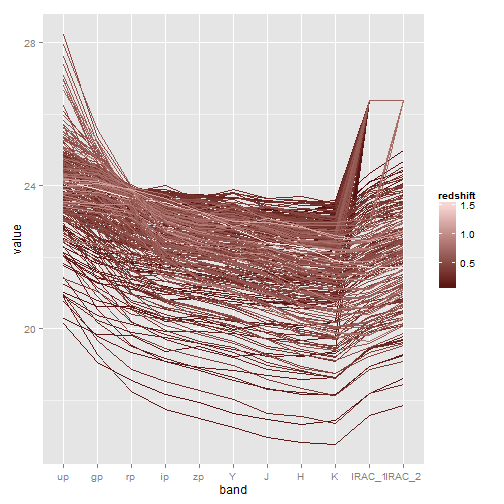Darker colours are low redshifts and lighter colors are higher redshifts. There appears to be a relation between the patterns and levels in the frequency bands and the redshifts. Notice the strange patterns in bands IRAC-1 and IRAC-2. Apparently there is something wrong with these bands for some of the objects. We do not remove or correct these values, but just leave them in.

## Principal components

The authors of the paper and the package suggest reducing the dimensionality of the feature space using Principal Component Analysis (PCA). A function is provided to achieve this, computeCombPCA(). This function essentially stacks the train and test sets and then conducts a PCA, using either PCAgrid() or prcomp(). In R, the code within a function is easily obtained by entering the function name without brackets:

``````CosmoPhotoz::computeCombPCA
``````
``````function (x, y, robust = TRUE)
{
if (!is.matrix(x) & !is.data.frame(x)) {
stop("Error in computeCombPCA :: x is nor a matrix neither a data frame. The code expects a matrix or data frame.")
}
if (!is.matrix(y) & !is.data.frame(y)) {
stop("Error in computeCombPCA :: y is nor a matrix neither a data frame. The code expects a matrix or data frame.")
}
XY <- rbind(x, y)
if (robust) {
XYPCA <- PCAgrid(XY, k = ncol(XY) - 1, scale = "mad",
X.PCA <- as.data.frame(XYPCA\$scores[1:nrow(x), ])
Y.PCA <- as.data.frame(XYPCA\$scores[(nrow(x) + 1):nrow(XY),
])
}
else {
XYPCA <- prcomp(XY, scale = TRUE)
XYPCA\$x <- XYPCA\$x[, 1:ncol(XY) - 1]
nn <- c()
for (i in 1:ncol(XYPCA\$x)) {
nn <- c(nn, paste("Comp.", i, sep = ""))
}
X.PCA <- as.data.frame(XYPCA\$x[1:nrow(x), ])
Y.PCA <- as.data.frame(XYPCA\$x[(nrow(x) + 1):nrow(XY),
])
names(X.PCA) <- nn
names(Y.PCA) <- nn
}
return(list(x = X.PCA, y = Y.PCA, PCsum = summary(XYPCA)))
}
<environment: namespace:CosmoPhotoz>
``````

Given that this function does nothing too fancy, we conduct the PCA directly using prcomp() because that gives us access to the factor loadings, which are unfortunately not returned by computeCombPCA(). The only thing we need to do is stack the train and test sets first.

``````PHAT0 = rbind(PHAT0train, PHAT0test)
myPCA = prcomp(PHAT0[,-1], scale = TRUE)
``````

Let's look at the results of the PCA. Of interest is the variance explained, and the factor loadings.

``````summary(myPCA)
``````
``````Importance of components:
PC1    PC2     PC3     PC4     PC5     PC6
Standard deviation     2.8905 1.4715 0.60362 0.22769 0.17636 0.11246
Proportion of Variance 0.7595 0.1968 0.03312 0.00471 0.00283 0.00115
Cumulative Proportion  0.7595 0.9564 0.98950 0.99421 0.99704 0.99819
PC7     PC8     PC9    PC10    PC11
Standard deviation     0.09414 0.06603 0.05802 0.04446 0.03657
Proportion of Variance 0.00081 0.00040 0.00031 0.00018 0.00012
Cumulative Proportion  0.99900 0.99939 0.99970 0.99988 1.00000
``````
``````myPCA\$rotation
``````
``````             PC1         PC2         PC3         PC4         PC5
up     0.1844886  0.51798612 -0.57123755 -0.51353462  0.23994070
gp     0.2653974  0.41775965 -0.23395834  0.39895314 -0.20376403
rp     0.3214594  0.23280431  0.01527489  0.49117882 -0.07516334
ip     0.3408146  0.08630742  0.14635438  0.01019729 -0.01534642
zp     0.3431729  0.02751705  0.16193598 -0.05804733 -0.17372129
Y      0.3430085 -0.00524629  0.17734887 -0.02325404  0.03983347
J      0.3425415 -0.05127861  0.17726695  0.01432890 -0.04136075
H      0.3376168 -0.11497440  0.19754407 -0.24711045  0.10248692
K      0.3326077 -0.15015441  0.21611996 -0.34415266  0.19088474
IRAC_1 0.2157248 -0.48416527 -0.49397990 -0.15471188 -0.65333696
IRAC_2 0.2283201 -0.47108891 -0.42243477  0.35805310  0.62270169
PC6         PC7         PC8         PC9        PC10
up      0.115083522 -0.04759433  0.05817588 -0.14442329 -0.10059476
gp     -0.443876164  0.33277194 -0.20721577  0.35835569  0.14046866
rp      0.691810916 -0.13599340 -0.12953851 -0.23627301  0.12087889
ip     -0.090228629 -0.71686267  0.17620306  0.44521320 -0.11124900
zp     -0.023319269  0.26736184  0.83194557 -0.08802721  0.16815589
Y      -0.493488054 -0.24013223 -0.21660548 -0.67677174  0.20595004
J       0.031707697  0.34692194 -0.09205073 -0.12662810 -0.76938657
H       0.009845866 -0.02690930 -0.23874413  0.25028952 -0.16698660
K       0.216899849  0.30998026 -0.26071805  0.22523444  0.50130091
IRAC_1  0.065798225 -0.08812288 -0.09950369 -0.02328286  0.03492527
IRAC_2 -0.080216381  0.02308953  0.15313750  0.03102311 -0.01033402
PC11
up     -0.013468323
gp      0.027737352
rp      0.104943245
ip     -0.301604694
zp      0.146692065
Y      -0.013794757
J      -0.329958056
H       0.783489496
K      -0.389999367
IRAC_1 -0.022086005
IRAC_2  0.006819349
``````

We learn from this that 5 components explain more than 99.5% of the variance. From the factor loadings we see that there are no obvious frequency bands that dominate the first few components. If there were, that would be an argument to use these bands specifically and not do PCA. The first PC is roughly an overall average. The second PC is a difference of the first half of the bands and the second half. The third PC contrasts the extreme bands and the middle bands. Together these three explain much of the variance. So, in this case, PCA seems well suited to reduce the dimension of the feature space. Elliot et al. choose to retain the first 6 components accounting for more than 99.8% of the variance in the data. Since we subsampled the training set, our results are not identical to those in the tutorial, but differences are minor. In what follows, we retain the first five components.

We prepare for modeling by appending the principal components to the data:

``````PHAT0train = cbind(PHAT0train,myPCA\$x[1:nrow(PHAT0train),])
PHAT0test = cbind(PHAT0test,myPCA\$x[(1+nrow(PHAT0train)):nrow(myPCA\$x),])
pccols = names(PHAT0train)[13:23]
``````

## Modeling and prediction

We start with two very simple models, as a reference for benchmarking.

``````mod1 = as.formula(paste("redshift ~ ",paste(bands,collapse=" + ")))
mod2 = as.formula(paste("redshift ~ ",paste(pccols[1:5],collapse=" + ")))
mod1
``````
``````redshift ~ up + gp + rp + ip + zp + Y + J + H + K + IRAC_1 +
IRAC_2
``````
``````mod2
``````
``````redshift ~ PC1 + PC2 + PC3 + PC4 + PC5
``````

The first model contains as predictors the magnitudes in the 11 wavelength bands directly. The second model contains the first five principal components. These simple models do not contain interactions. We consider these as linear models and fit them as follows.

``````fit1 = lm(mod1, PHAT0train)
fit2 = lm(mod2, PHAT0train)
``````
`````` 0.8611751
``````
``````summary(fit2)\$adj.r.squared
``````
`````` 0.7780046
``````
``````AIC(fit1)
``````
`````` -17025.79
``````
``````AIC(fit2)
``````
`````` -13051.84
``````

Of these two models, the first has a higher adjusted R squared, so it explains more of the variance than model 2. Since model 2 is of lower dimension this was to be expected. Looking at the AIC, which takes into account model complexity, the first model is again the best (lower values are better). Now we look at predictive accuracy to assess performance of the models.

``````pred1 = predict(fit1,PHAT0test)
pred2 = predict(fit2,PHAT0test)
RMSE = function(x) {
return(sqrt(mean((x - PHAT0test\$redshift)^2)))
}
RMSE(pred1)
``````
`````` 0.08752431
``````
``````RMSE(pred2)
``````
`````` 0.1075363
``````

We defined the RMSE() function to take the Root Mean Square Error. The RMSE of the first model is better (lower) than that of model 2. We can plot the predictions against the known values as follows.

``````qplot(PHAT0test\$redshift,pred1,geom="point") +
geom_abline(intercept=0, slope=1)
``````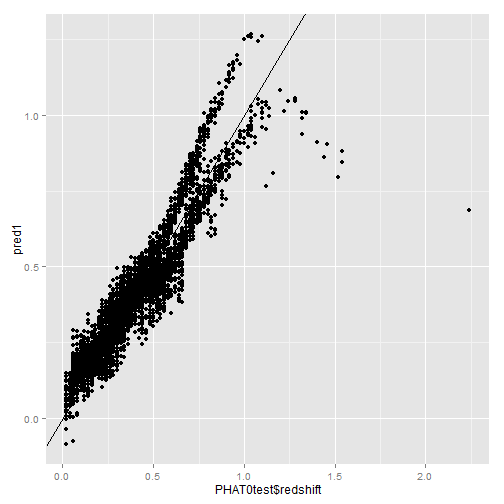``````qplot(PHAT0test\$redshift,pred2,geom="point") +
geom_abline(intercept=0, slope=1)
``````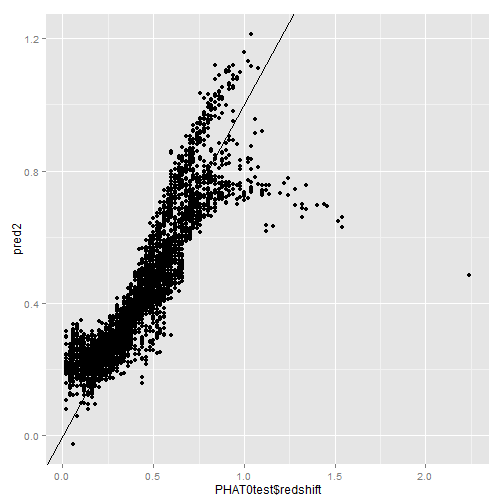We clearly see that the model specification seems to be wrong for redshifts higher than about 0.5. At these higher redshifts, there is a discrepancy between the predictions and the true values, for both models.

Now we turn to generalised linear models (GLMs). GLMs allow more flexibility in terms of the relation between de dependent variable and the predictors, and in terms of distributional assumptions. See Elliot et al. (2014) - mentioned above - for details.

We define the model proposed in the paper and fit it using the function provided in the package. We use 5 components rather than 6 since here these 5 explain more than 99.5% of the variance.

``````mod3 = redshift ~ poly(PC1,2) * poly(PC2,2) * PC3 * PC4 * PC5
fit3BayesGamma = glmTrainPhotoZ(PHAT0train, formula=mod3,
method="Bayesian", family="gamma")
fit3FreqGamma = glmTrainPhotoZ(PHAT0train, formula=mod3,
method="Frequentist", family="gamma")
qplot(fit3BayesGamma\$glmfit\$fitted.values,
fit3FreqGamma\$glmfit\$fitted.values,
geom="point")
``````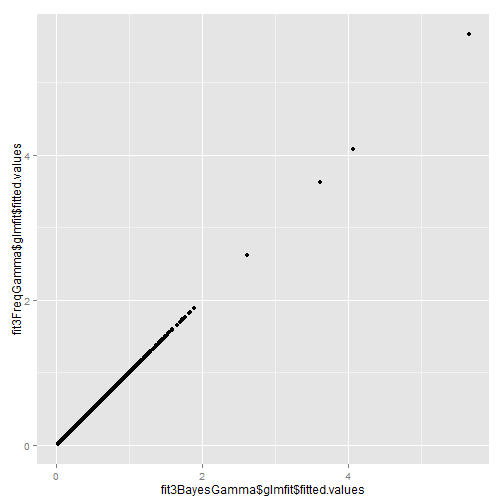Whether we do this in a frequentist or a bayesian setting makes no difference for the fitted values, as expected. It is really in terms of uncertainty quantification that the methods differ, but that aspect is not studied at present. Similarly, the predictions from both approaches are identical, so we only look at one of the two.

``````pred3BayesGamma = predict(fit3BayesGamma\$glmfit, PHAT0test, type = "response")
RMSE(pred3BayesGamma)
``````
`````` 0.07595157
``````
``````qplot(PHAT0test\$redshift,pred3BayesGamma,geom="point") +
geom_abline(intercept=0, slope=1)
``````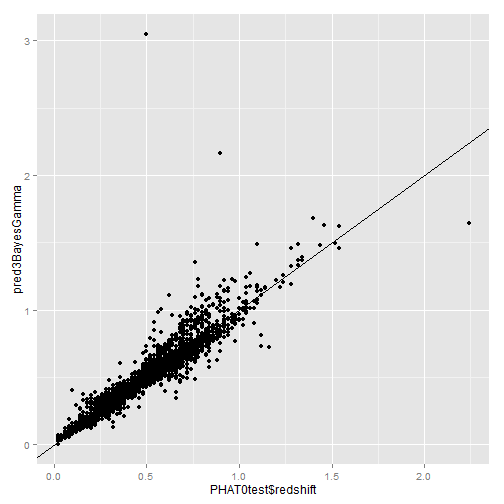OK, looking good. The RMSE is now smaller than for our initial models, and the predictions seem better when looking at the plot.

However, two things have changed, (i) the model is different and involves interaction terms, and (ii) a GLM model is used (Gamma with log link function). We now look at two alternatives: a linear model with the fancy formula, and a gamma model with the simple formula.

``````fit4 = glm(mod3, data=PHAT0train, family=gaussian)
fit5 = glmTrainPhotoZ(PHAT0train, formula=mod1,
method="Frequentist", family="gamma")
pred4 = predict(fit4, PHAT0test)
pred5 = predict(fit5\$glmfit, PHAT0test, type="response")
RMSE(pred4)
``````
`````` 0.03474182
``````
``````RMSE(pred5)
``````
`````` 0.1591499
``````

Fitting a simple linear model (fit4) using the model formula from the paper (involving the principal components, second order effects and interactions) gives a RMSE well below that using a Gamma model! Using the Gamma with the simple model (fit5) is not a good idea, judging by the RMSE. Let's look at the predictions of the linear model (LM) in comparison to those of the GLM proposed in the paper.

``````X = data.frame(true = rep(PHAT0test\$redshift,2),
predicted = c(pred3BayesGamma, pred4),
method = rep(c("GLM","LM"), each=length(pred4)))
qplot(x=true, y=predicted, data=X,
geom="point", colour=method,size=I(0.8)) +
geom_abline(intercept=0, slope=1) +
scale_colour_manual(values=c("red", "blue"))
``````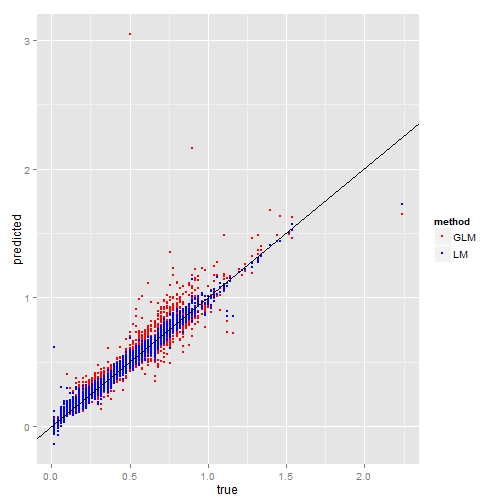The LM predictions (in blue) look pretty good. In fact, better than the GLM predictions using the Gamma model (in red).

The CosmoPhotoz package comes with some additional functions. The function computeDiagPhotoZ() gives some useful diagnostics. We look at the diagnostics of the approach presented in Elliot et al. (2014) and those of using a linear model instead. We focus on the percentage of outliers as defined in the paper.

``````computeDiagPhotoZ(pred3BayesGamma, PHAT0test\$redshift)\$outliers
``````
`````` "0.85%"
``````
``````computeDiagPhotoZ(pred4, PHAT0test\$redshift)\$outliers
``````
`````` "0.12%"
``````

Also in terms of outliers is the linear model better than the GLM.

The CosmoPhotoz package has a function plotDiagPhotoZ() which can produce different plots of the residuals. We do not explore this further here, as we want to concentrate on diagnostic plots. The package boot comes with a function glm.diag() which calculates different residues. Of interest here are the standardized deviance residuals, which should be standard-normally distributed if model assumptions hold. The following code obtains these residuals for both the GLM and LM approaches and makes qq-plots to assess the distributional characteristics.

``````rdGlm = glm.diag(fit3FreqGamma\$glmfit)\$rd
rdLm = glm.diag(fit4)\$rd
qplot(sample = rdGlm, dist = qnorm,
dparams = list(mean=0,sd=1),
main="GLM residues") +
geom_abline(intercept=0, slope=1)
``````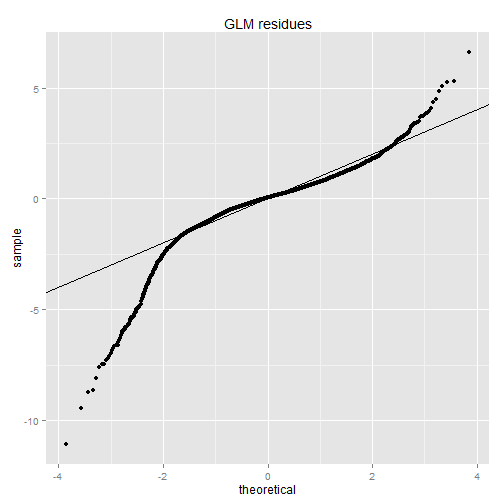``````qplot(sample = rdLm, dist = qnorm,
dparams = list(mean=0,sd=1),
main="LM residues") +
geom_abline(intercept=0, slope=1)
``````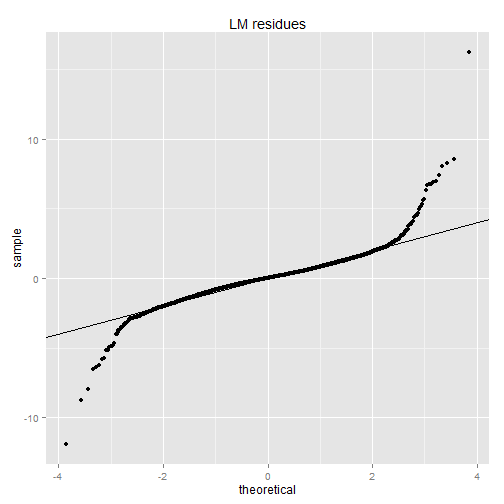From the above plots, we see that the standardized deviance residues from the linear model are somewhat closer to normal than the residues from the GLM, in particular at the lower tail. Note that the boot package has a function glm.diag.plots() allowing even more diagnostic assessments.

## Conclusions

We explored in fairly great detail the functionality of the CosmoPhotoz package for R. We used a data set that comes with the package. We repeated much of the analysis provided in a tutorial and a paper by the authors of the package (see top for references and links), and expanded upon it in various ways.

It is really nice that the authors provide their analysis routines as a package. This makes their proposed procedures a lot easier to understand and to reproduce. For amateurs and hobbyists interested in astrostatistics, such a package provides an excellent point of entry into the field. We hope that the present document clarifies the methods further.

While putting this material together we found that a linear model seems to perform better than the gamma model proposed by the authors of the package and paper, at least on the one data set investigated here, which was produced through simulation. This signals the need for careful model selection and checking. In fact, no serious model selection has been conducted here, as many more models than the ones analysed here are thinkable.